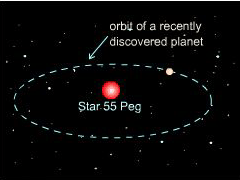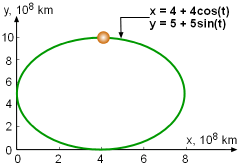Ch 10. Parametric Equations Multimedia Engineering Math ParametricEquations Tangentsand Areas Arc Length Surface Area
 Chapter 1. Limits 2. Derivatives I 3. Derivatives II 4. Mean Value 5. Curve Sketching 6. Integrals 7. Inverse Functions 8. Integration Tech. 9. Integrate App. 10. Parametric Eqs. 11. Polar Coord. 12. Series Appendix Basic Math Units Search eBooks Dynamics Fluids Math Mechanics Statics Thermodynamics Author(s): Hengzhong Wen Chean Chin Ngo Meirong Huang Kurt Gramoll ©Kurt GramollMATHEMATICS - CASE STUDY IntroductionProblem DiagramTop View of the Planet's Orbital Path Dr. Smith, a scientist at the Jetway Propulsion Laboratory, has recently discovered a planet orbit around a distant solar-type star named 55 Peg. The planet's path is found similar to an ellipse as shown in the figure. For a report on the new planet, he needs to estimate the distance traveled by the planet during a complete orbit. Questions Determine the arc length of one complete orbit. Approach The parametric equations for the ellipse are given as x = 4 + 4cos(t) and y = 5 + 5sin(t) for 0 ≤ t ≤ 2π where x and y are in terms of 108 km. Use Simpson's Rule to evaluate the integral if necessary.